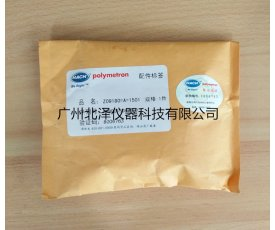位置： 仪器谱首页 > > 测量板Z09180=A=1501
位置： 仪器谱首页 > > 测量板Z09180=A=1501

## 测量板Z09180=A=1501(哈希)

#### 广州北泽仪器科技有限公司CPU板带显示Z09125=A=1001 电流测量板Z09180=A=1501 显示屏Z09182=A=6211 CPU主板Z09182=A=6211 溶解氧电极Z09182=A=1000 10m电极电缆Z09180=A=8010 溶解氧膜Z09185=A=3500

1 211194 奥立龙 8.4
2 800 瑞士万通 8.1
3 3ESTC15 合肥科晶 7.7
Z09180=A=1501